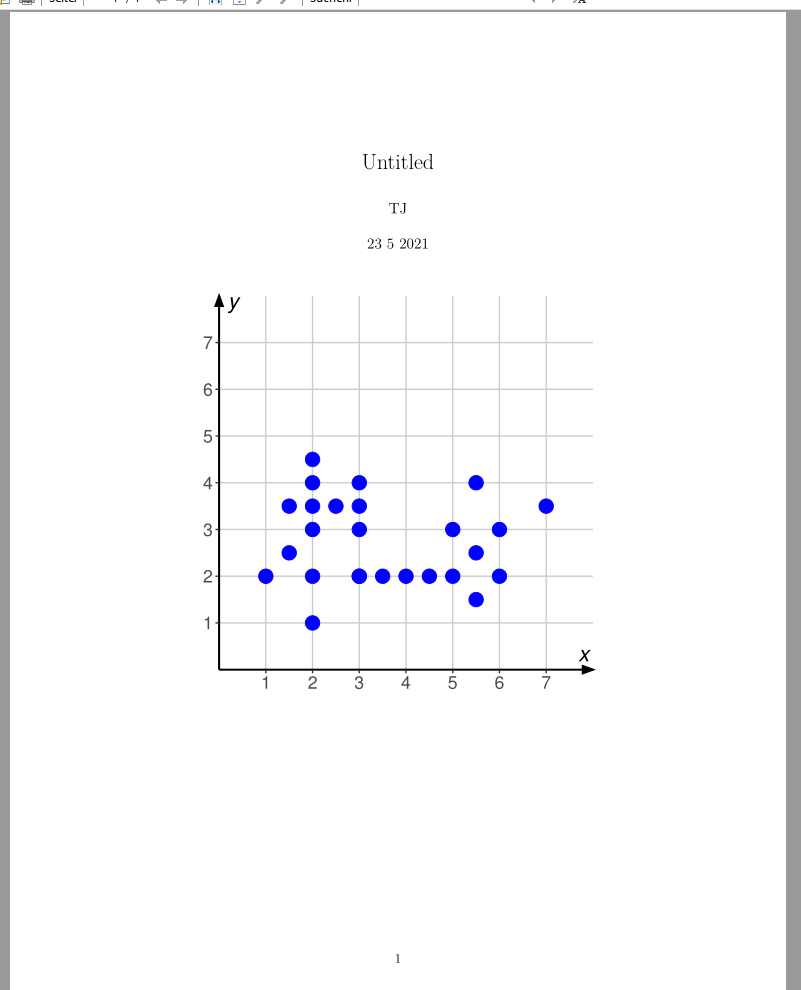# Graphics error while knitting from Rmarkdown to PDF

I have a Rmarkdown file that uses 3 libraries: `extrafont`,`ggplot2`,`dplyr`. I have to generate a PDF after knitting this document. But it gives me an error as below:

``````Quitting from lines 21-52 (A6.3.Rmd)
Error in grid.Call.graphics(C_text, as.graphicsAnnot(x\$label), x\$x, x\$y,  :
invalid font type
Calls: <Anonymous> ... drawDetails -> drawDetails.text -> grid.Call.graphics
In addition: There were 50 or more warnings (use warnings() to see the first 50)
``````

This is not the case when I knit to HTML. It works fine.

Below is the code:

``````library(ggplot2)
library(extrafont)
library(dplyr)

df <- data.frame(x = c(1,2,2,3,3.5,4,4.5,5,5.5,6,1.5,2,2,2,2,1.5,2.5,3,3,3,3,5.5,5,6,5.5,7)
,y = c(2,1,2,2,2,2,2,2,1.5,2,2.5,3,3.5,4,4.5,3.5,3.5,2,3,3.5,4,2.5,3,3,4,3.5)
)

# helper dataframe for axis
df_arrow <- data.frame(x = c(0, 0),
y = c(0, 0),
xend = c(0, 8),
yend = c(8, 0))

ggplot(df,aes(x, y)) +
geom_point(colour = "blue", size = 5)+
scale_x_continuous(breaks = 1:7, expand = expansion(add = c(0, 1)))+
scale_y_continuous(breaks = 1:7, expand = expansion(add = c(0, 1)))+
coord_fixed(xlim = c(0, 7), ylim = c(0, 7), clip = "off")+
geom_segment(data = df_arrow, aes(x = x, xend = xend, y = y, yend = yend), size = 0.75, colour = "black",
arrow = arrow(angle = 20, length = unit(3, "mm"), ends = "last", type = "closed"), linejoin = "mitre") +
annotate("text", x = c(7.8, 0.3), y = c(0.3, 7.8), label = c("italic(x)", "italic(y)"), parse = TRUE, size = 6,  family = "Arial Narrow")+
labs(x = NULL,
y = NULL)+
theme_bw()+
theme(panel.grid.major = element_line(colour = "gray80"),
panel.grid.minor = element_blank(),
panel.border = element_blank(),
axis.ticks.length = unit(1, "mm"),
text = element_text(size = 18,  family = "Arial Narrow"))
``````

And also there are 2 more similar blocks that give slightly different plots.

Not sure if it is with this `extrafont` library that I’m working with.

when I inspect this error it is with this code block that it specifies.

## Solution

The issue is with `family = "Arial Narrow"`

If you remove `family = "Arial Narrow"` in these two lines:

1. `annotate("text", x = c(7.8, 0.3), y = c(0.3, 7.8), label = c("italic(x)", "italic(y)"), parse = TRUE, size = 6, family = "Arial Narrow")+`
2. `text = element_text(size = 18, family = "Arial Narrow"))`

The knitting to pdf will work: